Smartick is an online platform for children to master math in only 15 minutes a day

# I want to learn about: Number & Operations – Fractions

Content about fractions in elementary mathematics. Exercises, tutorials, problems, and teaching resources for fractions in elementary. Here you will find posts about fractions to make learning mathematics easier and more fun. ido generado sobre fracciones de matemáticas de primaria.

Jan11

## Learning How to Subtract Fractions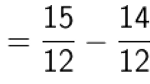In this post, we are going to see how to subtract fractions, but for that, you first have to know what the denominator and numerator of a fraction are. The numerator is the number that is written on top of the fraction and the denominator is the number written on the bottom of the fraction. […]

Oct25

## Learn about Fractions: Halves, Thirds and Fourths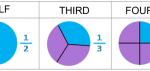Welcome to Smartick’s blog! Today, we are going to talk about the fractions that we use on a daily basis. I’m sure that you’ve heard of these expressions, and others like them on more than one occasion: Fractions “I have half of a sandwich…”     “You’ve eaten a third of your chocolate!”   “Could […]

Oct10

## Singapore Bars Applied to Fractions

In today’s post, we are going to learn how to apply Singapore bars to problems with fractions. As we know, we can be asked for various amounts in problems with fractions….so let’s look at a few examples so it is very clear to us! Mind you, fractional numbers have become easy for us so they […]

Sep29

## Practice Estimating Fractions with Examples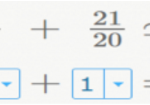Today we will practice estimating fractions! We’re going to look at the types of problems below: Problem 1:  Estimating Fractions. To get a better idea, we’re going to represent the fractions with bars. Let’s start with the first fraction. We can see that the fraction is much closer to 0 than it is to 1. If […]

Aug29

## Examples of Equivalent FractionsWelcome back to the Smartick blog! Do you know what equivalent fractions are? After reading this post with examples of equivalent fractions, you’ll be an expert. Equivalent fractions are fractions that represent the same amount, even though they may appear to be different. Let’s look at this example: Do you think these fractions represent the […]

Jul28

## Equivalent Fractions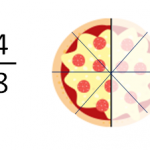What are equivalent fractions? In this post, we are going to understand what equivalent fractions are and how you can find out what fractions are equivalent. Equivalent fractions are fractions that represent the same quantity. For example, which of the following do you believe will be the biggest? What have you figured out? We are […]

Jul15

## Understand What a Fraction Is and When It Is Used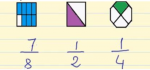With this post, we are going to understand the notion of a fraction as a part of a whole, as a division of natural numbers and its position on the number line. What is a fraction? A fraction represents the number of parts that we have of a whole that is divided into equal parts. Fractions […]